Intermediate Algebra 2e

# Key Concepts

### Key Concepts

#### 11.1Distance and Midpoint Formulas; Circles

• Distance Formula: The distance d between the two points $(x1,y1)(x1,y1)$ and $(x2,y2)(x2,y2)$ is
$d=(x2−x1)2+(y2−y1)2d=(x2−x1)2+(y2−y1)2$
• Midpoint Formula: The midpoint of the line segment whose endpoints are the two points $(x1,y1)(x1,y1)$ and $(x2,y2)(x2,y2)$ is
$(x1+x22,y1+y22)(x1+x22,y1+y22)$

To find the midpoint of a line segment, we find the average of the x-coordinates and the average of the y-coordinates of the endpoints.
• Circle: A circle is all points in a plane that are a fixed distance from a fixed point in the plane. The given point is called the center, $(h,k),(h,k),$ and the fixed distance is called the radius, r, of the circle.
• Standard Form of the Equation a Circle: The standard form of the equation of a circle with center, $(h,k),(h,k),$ and radius, r, is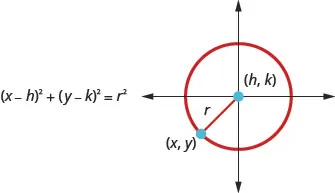• General Form of the Equation of a Circle: The general form of the equation of a circle is
$x2+y2+ax+by+c=0x2+y2+ax+by+c=0$

#### 11.2Parabolas

• Parabola: A parabola is all points in a plane that are the same distance from a fixed point and a fixed line. The fixed point is called the focus, and the fixed line is called the directrix of the parabola.
Vertical Parabolas
General form
$y=ax2+bx+cy=ax2+bx+c$
Standard form
$y=a(x−h)2+ky=a(x−h)2+k$
Orientation $a>0a>0$ up; $a<0a<0$ down $a>0a>0$ up; $a<0a<0$ down
Axis of symmetry $x=−b2ax=−b2a$ $x=hx=h$
Vertex Substitute $x=−b2ax=−b2a$ and
solve for y.
$(h,k)(h,k)$
y- intercept Let $x=0x=0$ Let $x=0x=0$
x-intercepts Let $y=0y=0$ Let $y=0y=0$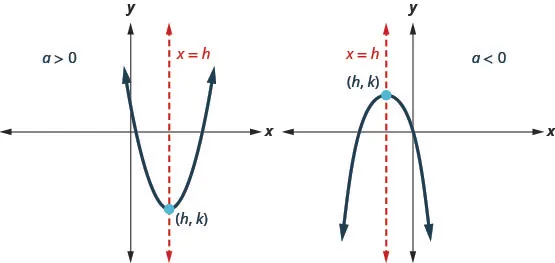• How to graph vertical parabolas $(y=ax2+bx+c(y=ax2+bx+c$ or $f(x)=a(x−h)2+k)f(x)=a(x−h)2+k)$ using properties.
1. Step 1. Determine whether the parabola opens upward or downward.
2. Step 2. Find the axis of symmetry.
3. Step 3. Find the vertex.
4. Step 4. Find the y-intercept. Find the point symmetric to the y-intercept across the axis of symmetry.
5. Step 5. Find the x-intercepts.
6. Step 6. Graph the parabola.

Horizontal Parabolas
General form
$x=ay2+by+cx=ay2+by+c$
Standard form
$x=a(y−k)2+hx=a(y−k)2+h$
Orientation $a>0a>0$ right; $a<0a<0$ left $a>0a>0$ right; $a<0a<0$ left
Axis of symmetry $y=−b2ay=−b2a$ $y=ky=k$
Vertex Substitute $y=−b2ay=−b2a$ and
solve for x.
$(h,k)(h,k)$
y-intercepts Let $x=0x=0$ Let $x=0x=0$
x-intercept Let $y=0y=0$ Let $y=0y=0$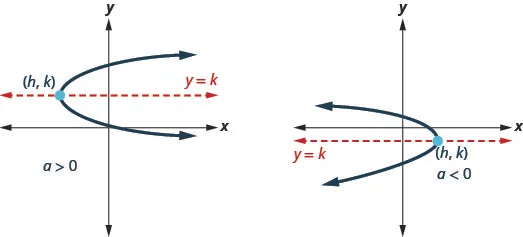• How to graph horizontal parabolas $(x=ay2+by+c(x=ay2+by+c$ or $x=a(y−k)2+h)x=a(y−k)2+h)$ using properties.
1. Step 1. Determine whether the parabola opens to the left or to the right.
2. Step 2. Find the axis of symmetry.
3. Step 3. Find the vertex.
4. Step 4. Find the x-intercept. Find the point symmetric to the x-intercept across the axis of symmetry.
5. Step 5. Find the y-intercepts.
6. Step 6. Graph the parabola.

#### 11.3Ellipses

• Ellipse: An ellipse is all points in a plane where the sum of the distances from two fixed points is constant. Each of the fixed points is called a focus of the ellipse.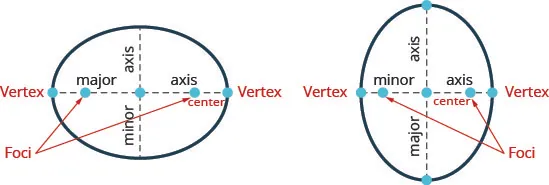If we draw a line through the foci intersects the ellipse in two points—each is called a vertex of the ellipse.
The segment connecting the vertices is called the major axis.
The midpoint of the segment is called the center of the ellipse.
A segment perpendicular to the major axis that passes through the center and intersects the ellipse in two points is called the minor axis.
• Standard Form of the Equation an Ellipse with Center $(0,0):(0,0):$ The standard form of the equation of an ellipse with center $(0,0),(0,0),$ is
$x2a2+y2b2=1x2a2+y2b2=1$

The x-intercepts are $(a,0)(a,0)$ and $(−a,0).(−a,0).$
The y-intercepts are $(0,b)(0,b)$ and $(0,−b).(0,−b).$
• How to an Ellipse with Center $(0,0)(0,0)$
1. Step 1. Write the equation in standard form.
2. Step 2. Determine whether the major axis is horizontal or vertical.
3. Step 3. Find the endpoints of the major axis.
4. Step 4. Find the endpoints of the minor axis
5. Step 5. Sketch the ellipse.
• Standard Form of the Equation an Ellipse with Center $(h,k):(h,k):$ The standard form of the equation of an ellipse with center $(h,k),(h,k),$ is
$(x−h)2a2+(y−k)2b2=1(x−h)2a2+(y−k)2b2=1$

When $a>b,a>b,$ the major axis is horizontal so the distance from the center to the vertex is a.
When $b>a,b>a,$ the major axis is vertical so the distance from the center to the vertex is b.

#### 11.4Hyperbolas

• Hyperbola: A hyperbola is all points in a plane where the difference of their distances from two fixed points is constant.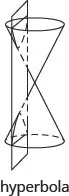Each of the fixed points is called a focus of the hyperbola.
The line through the foci, is called the transverse axis.
The two points where the transverse axis intersects the hyperbola are each a vertex of the hyperbola.
The midpoint of the segment joining the foci is called the center of the hyperbola.
The line perpendicular to the transverse axis that passes through the center is called the conjugate axis.
Each piece of the graph is called a branch of the hyperbola.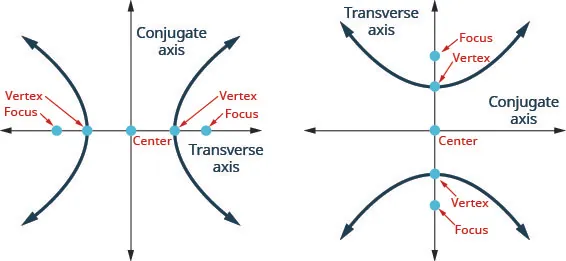Standard Forms of the Equation a Hyperbola with Center $(0,0)(0,0)$
$x2a2−y2b2=1x2a2−y2b2=1$ $y2a2−x2b2=1y2a2−x2b2=1$
Orientation Transverse axis on the x-axis.
Opens left and right
Transverse axis on the y-axis.
Opens up and down
Vertices $(−a,0),(−a,0),$ $(a,0)(a,0)$ $(0,−a),(0,−a),$ $(0,a)(0,a)$
x-intercepts $(−a,0),(−a,0),$ $(a,0)(a,0)$ none
y-intercepts none $(0,−a)(0,−a)$, $(0,a)(0,a)$
Rectangle Use $(±a,0)(±a,0)$ $(0,±b)(0,±b)$ Use $(0,±a)(0,±a)$ $(±b,0)(±b,0)$
asymptotes $y=bax,y=bax,$ $y=−baxy=−bax$ $y=abx,y=abx,$ $y=−abxy=−abx$
• How to graph a hyperbola centered at $(0,0).(0,0).$
1. Step 1. Write the equation in standard form.
2. Step 2. Determine whether the transverse axis is horizontal or vertical.
3. Step 3. Find the vertices.
4. Step 4. Sketch the rectangle centered at the origin intersecting one axis at $±a±a$ and the other at $±b.±b.$
5. Step 5. Sketch the asymptotes—the lines through the diagonals of the rectangle.
6. Step 6. Draw the two branches of the hyperbola.

Standard Forms of the Equation a Hyperbola with Center $(h,k)(h,k)$
$(x−h)2a2−(y−k)2b2=1(x−h)2a2−(y−k)2b2=1$ $(y−k)2a2−(x−h)2b2=1(y−k)2a2−(x−h)2b2=1$
Orientation Transverse axis is horizontal.
Opens left and right
Transverse axis is vertical.
Opens up and down
Center $(h,k)(h,k)$ $(h,k)(h,k)$
Vertices a units to the left and right of the center a units above and below the center
Rectangle Use a units left/right of center
b units above/below the center
Use a units above/below the center
b units left/right of center
• How to graph a hyperbola centered at $(h,k).(h,k).$
1. Step 1. Write the equation in standard form.
2. Step 2. Determine whether the transverse axis is horizontal or vertical.
3. Step 3. Find the center and $a,b.a,b.$
4. Step 4. Sketch the rectangle centered at $(h,k)(h,k)$ using $a,b.a,b.$
5. Step 5. Sketch the asymptotes—the lines through the diagonals of the rectangle. Mark the vertices.
6. Step 6. Draw the two branches of the hyperbola.

Conic Characteristics of $x2-x2-$ and $y2-y2-$ terms Example
Parabola Either $x2x2$ OR $y2.y2.$ Only one variable is squared. $x=3y2−2y+1x=3y2−2y+1$
Circle $x2-x2-$ and $y2-y2-$ terms have the same coefficients $x2+y2=49x2+y2=49$
Ellipse $x2-x2-$ and $y2-y2-$ terms have the same sign, different coefficients $4x2+25y2=1004x2+25y2=100$
Hyperbola $x2-x2-$ and $y2-y2-$ terms have different signs, different coefficients $25y2−4x2=10025y2−4x2=100$

#### 11.5Solve Systems of Nonlinear Equations

• How to solve a system of nonlinear equations by graphing.
1. Step 1. Identify the graph of each equation. Sketch the possible options for intersection.
2. Step 2. Graph the first equation.
3. Step 3. Graph the second equation on the same rectangular coordinate system.
4. Step 4. Determine whether the graphs intersect.
5. Step 5. Identify the points of intersection.
6. Step 6. Check that each ordered pair is a solution to both original equations.
• How to solve a system of nonlinear equations by substitution.
1. Step 1. Identify the graph of each equation. Sketch the possible options for intersection.

2. Step 2. Solve one of the equations for either variable.
3. Step 3. Substitute the expression from Step 2 into the other equation.
4. Step 4. Solve the resulting equation.
5. Step 5. Substitute each solution in Step 4 into one of the original equations to find the other variable.
6. Step 6. Write each solution as an ordered pair.
7. Step 7. Check that each ordered pair is a solution to both original equations.
• How to solve a system of equations by elimination.
1. Step 1. Identify the graph of each equation. Sketch the possible options for intersection.
2. Step 2. Write both equations in standard form.
3. Step 3. Make the coefficients of one variable opposites.
Decide which variable you will eliminate.
Multiply one or both equations so that the coefficients of that variable are opposites.
4. Step 4. Add the equations resulting from Step 3 to eliminate one variable.
5. Step 5. Solve for the remaining variable.
6. Step 6. Substitute each solution from Step 5 into one of the original equations. Then solve for the other variable.
7. Step 7. Write each solution as an ordered pair.
8. Step 8. Check that each ordered pair is a solution to both original equations.
Order a print copy

As an Amazon Associate we earn from qualifying purchases.